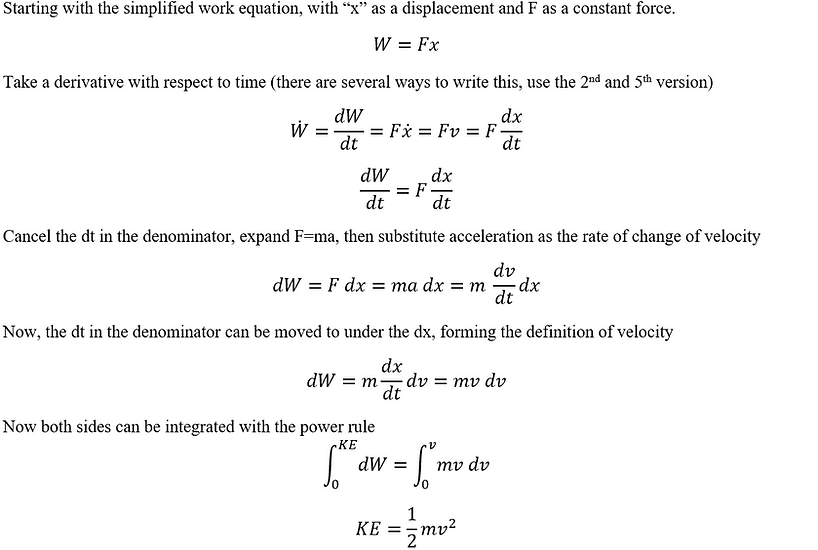top of page

## Types of Energy

Energy is a concept we use in common language to describe moving things, burning things, and much more. Below is a list of types of energy and power used in engineering.There are 2 common ways to write the Conservation of Energy equations, Work added to the initial, or subtracted from the final energy as shown below with "E" as the total energy stored in the initial or final state. With simple algebra, they can be proven to be the same thing, but different textbooks and professors may insist on one form as the "correct" setup. In my experience, The 1st is more common in engineering classes and chemistry, and Physics professors use the 2nd. Conceptually the 1st is simple, where if work is put into the system, the final value is bigger. The 2nd is different, if the work is changing with respect to time, any 2 points can be set equal to another as shown in the 2nd problem in Work energy Block on a Slope. Because the work done by friction is always negative, the extra sign cancells in the 2nd equation; for an object sliding to a stop, think of the friction term getting larger with time an the other energy (probably kinetic) shrinking until it comes to a stop.Let's go through each of these in order.

Applied work uses the equation derived here, for work done by a constant force, this is used most often with friction and applied forces like somebody pulling or pushing an object. If a question asks how much work is done by a force, use this equation. The cos(theta) can change the sign of the work done. When towing a trailer on flat ground, the towing force is in the same direction as the motion, so cos(0) = 1 and W = Fd. In the same example, the weight of the trailer is pointed down, 90deg from the direction of motion, so cos(90) = 0, W = 0. Friction and air resistance want to slow down the trailer, pointing opposite the direction of motion, so cos(180) = -1 and W = -Fd.

Kinetic energy is the amount of work required to accelerate an object from rest to a given velocity (derivation below). KE is independent of direction; for example, a car on a banked turn can maintain the same speed while changing direction. The KE equation and Applied work equation can be rearranged to make the 5th kinematic equation.Rotational kinetic energy (RKE) has the same derivation as linear KE but uses the angular kinematics notation. Any rotating object has RKE, but rolling objects have both KE and RKE. If the object rolls without slipping, the velocities are related using v=omega*r.

Gravitational potential energy (PE) is simply the work equation for gravity over short distances, the d*cos(theta) can be substituted for the vertical displacement or change in height "h" and the force of gravity is "m*g". Set the lowest position in the problem as 0 PE, and measure the height relative to any other points of interest.

Spring Potential energy is the work held inside a compressed spring. Hooke's law for springs (a linear function) can be integrated with respect to distance from the neutral position to get the work function (quadratic) shown in the table above. Keep in mind that the energy is measured relative to some point, usually the neutral position. If the position of the spring changes during the problem, subtract the energy after plugging each position into the formula; do NOT subtract the positions first. For example, the same energy is contained within a spring when it's compressed some distance or stretched the same distance; there is no negative energy in the spring.

Far from the surface of the earth, lower gravity makes the weight non-constant so an integral is needed to find the (GPE). Because of the inverse "r", we cannot measure relative to the center of the Earth without a divide by zero so GPE is 0 at an infinite distance and negative anywhere closer. That way, a higher point has a less negative potential so the signs make sense.

bottom of page